# Sawhorse angles:height

I saw these simple sawhorses at a construction site and I’ve been wanting to make a pair for myself, but I’m not quite sure how to get the numbers I’m lookin for

I would want the footprint to be 36" W
I imagine the angle of the legs to be something like 15º
total height would be 36"

the question is: how long do i cut the legs?

I’m sure plenty of people around here have a quick “just do this” answer. I guess I could just make it in sketchup till it makes sense, but I’m sure there’s a formula that I can rememberize to make it easier

If you just want the legs to be triangular, i.e. not splaying to the left and right in that picture, then you’d just need to calculate the hypotenuse of the triangle, example: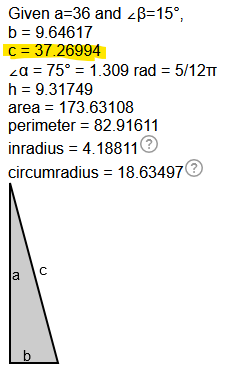Alternatively if you do want them to splay towards and away and be angled in both directions i.e.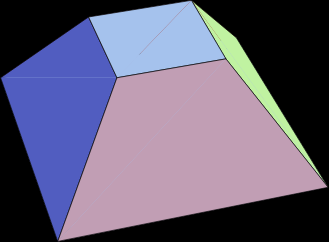you’d need to calculate the diagonal that starts in the middle of the base footprint and ends up in a corner. Then subtract the same diagonal for the top footprint. Meaning you’d need the other dimension of the base footprint, and both dimensions of the top footprint as well. If you wanted the footprint to be 36"x10" at the base, and form a rectangle at the top that is 6"x24", for example, then the center to corner distance of the base would would be: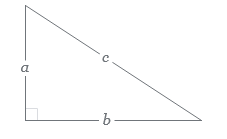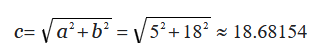and the top would be: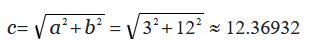Take the difference, and make that the new “b”, with the “a” being the desired height,and the leg length would then be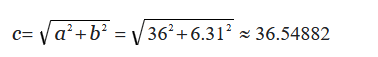So between ~36.5 and ~37.25 should get you to where you wanna be.

In the second example, the angle wouldn’t be exactly 15 degrees, but somewhere near. It’s probably better to just decide your top and bottom footprints and then use whatever length it spits out. If you wanted it to be exactly 15 degrees, then it would be just a little more complicated cause you’d have to use some more trigonometry. You can also extend the top to whatever length or width you want, that’s just uses the footprint of where the legs would meet the top.

Sorry for making this more complicated than it needed to be, hopefully it helps

2 Likes

Ok simpler solution: if you know you want it to be 36" tall and for the legs to splay, for example, 30 degrees to the left and 15 degrees towards you, then you can just do one after the other

cos(30 degrees) = 36/x
x=41.569"

cos(15 degrees) = 41.569/y
y=43.0354"

So your leg length would be 43". Just sub your desired angles and height to get leg length

2 Likes
1 Like